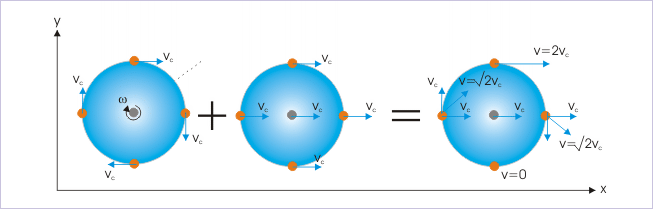# 11.15: Rotational Kinematics

•• Boundless
• Boundless
$$\newcommand{\vecs}{\overset { \rightharpoonup} {\mathbf{#1}} }$$ $$\newcommand{\vecd}{\overset{-\!-\!\rightharpoonup}{\vphantom{a}\smash {#1}}}$$$$\newcommand{\id}{\mathrm{id}}$$ $$\newcommand{\Span}{\mathrm{span}}$$ $$\newcommand{\kernel}{\mathrm{null}\,}$$ $$\newcommand{\range}{\mathrm{range}\,}$$ $$\newcommand{\RealPart}{\mathrm{Re}}$$ $$\newcommand{\ImaginaryPart}{\mathrm{Im}}$$ $$\newcommand{\Argument}{\mathrm{Arg}}$$ $$\newcommand{\norm}{\| #1 \|}$$ $$\newcommand{\inner}{\langle #1, #2 \rangle}$$ $$\newcommand{\Span}{\mathrm{span}}$$ $$\newcommand{\id}{\mathrm{id}}$$ $$\newcommand{\Span}{\mathrm{span}}$$ $$\newcommand{\kernel}{\mathrm{null}\,}$$ $$\newcommand{\range}{\mathrm{range}\,}$$ $$\newcommand{\RealPart}{\mathrm{Re}}$$ $$\newcommand{\ImaginaryPart}{\mathrm{Im}}$$ $$\newcommand{\Argument}{\mathrm{Arg}}$$ $$\newcommand{\norm}{\| #1 \|}$$ $$\newcommand{\inner}{\langle #1, #2 \rangle}$$ $$\newcommand{\Span}{\mathrm{span}}$$$$\newcommand{\AA}{\unicode[.8,0]{x212B}}$$

learning objectives

• Distinguish the two different motions in which rolling without slipping is broken down

Rolling without slipping generally occurs when an object rolls without skidding. To relate this to a real world phenomenon, we can consider the example of a wheel rolling on a flat, horizontal surface.

Connecting Linear and Rotational Motion! Rolling without Slipping!: How fast does the axle of a bike wheel move? How fast does the BOTTOM of a wheel move?

Rolling without slipping can be better understood by breaking it down into two different motions: 1) Motion of the center of mass, with linear velocity v (translational motion); and 2) rotational motion around its center, with angular velocity w.Rolling Motion: Rolling motion is a combination of rotational and translational motion.

When an object is rolling on a plane without slipping, the point of contact of the object with the plane does not move. If we imagine a wheel moving forward by rolling on a plane at speed v, it must also be rotating about its axis at an angular speed ωω since it is rolling.

The object’s angular velocity ωω is directly proportional to its velocity, because as we know, the faster we are driving in a car, the faster the wheels have to turn. So, to determine the exact relationship between linear velocity and angular velocity, we can imagine the scenario in which the wheel travels a distance of x while rotating through an angle θ.Rolling Without Slipping: A body rolling a distance of x on a plane without slipping.

In mathematical terms, the length of the arc is equal to the angle of the segment multiplied by the object’s radius, R. Therefore, we can say that the length of the arc of the wheel that has rotated an angle θ, is equal to Rθ. Furthermore, since the wheel is in constant contact with the ground, the length of the arc correlating to the angle is also equal to x. Therefore,

$\mathrm{x=Rθ}$

Since xx and θθ depend on time, we can take the derivative of both sides to obtain:

$\mathrm{\dfrac{dx}{dt}=R\dfrac{dθ}{dt}}$

where $$\mathrm{\frac{dx}{dt}}$$ is equal to the linear velocity $$\mathrm{v}$$, and $$\mathrm{\frac{dθ}{dt}}$$ is equal to the angular velocity ωω. We can then simplify this equation to:

$\mathrm{v=ωR}$

## Key Points

• Rolling without slipping can be better understood by breaking it down into translational motion and rotational motion.
• When an object is rolling on a plane without slipping, the point of contact of the object with the plane does not move.
• A rolling object’s velocity v is directly related to its angular velocity $$\mathrm{ω}$$, and is mathematically expressed as $$\mathrm{v=ωR}$$, where $$\mathrm{R}$$ is the object’s radius and $$\mathrm{v}$$ is its linear velocity.

## Key Terms

• angular velocity: A vector quantity describing the motion of an object in circular motion; its magnitude is equal to the angular speed (ωω) of the particle, and the direction is perpendicular to the plane of its circular motion.
• linear velocity: A vector quantity that denotes the rate of change of position with respect to time of the object’s center of mass.